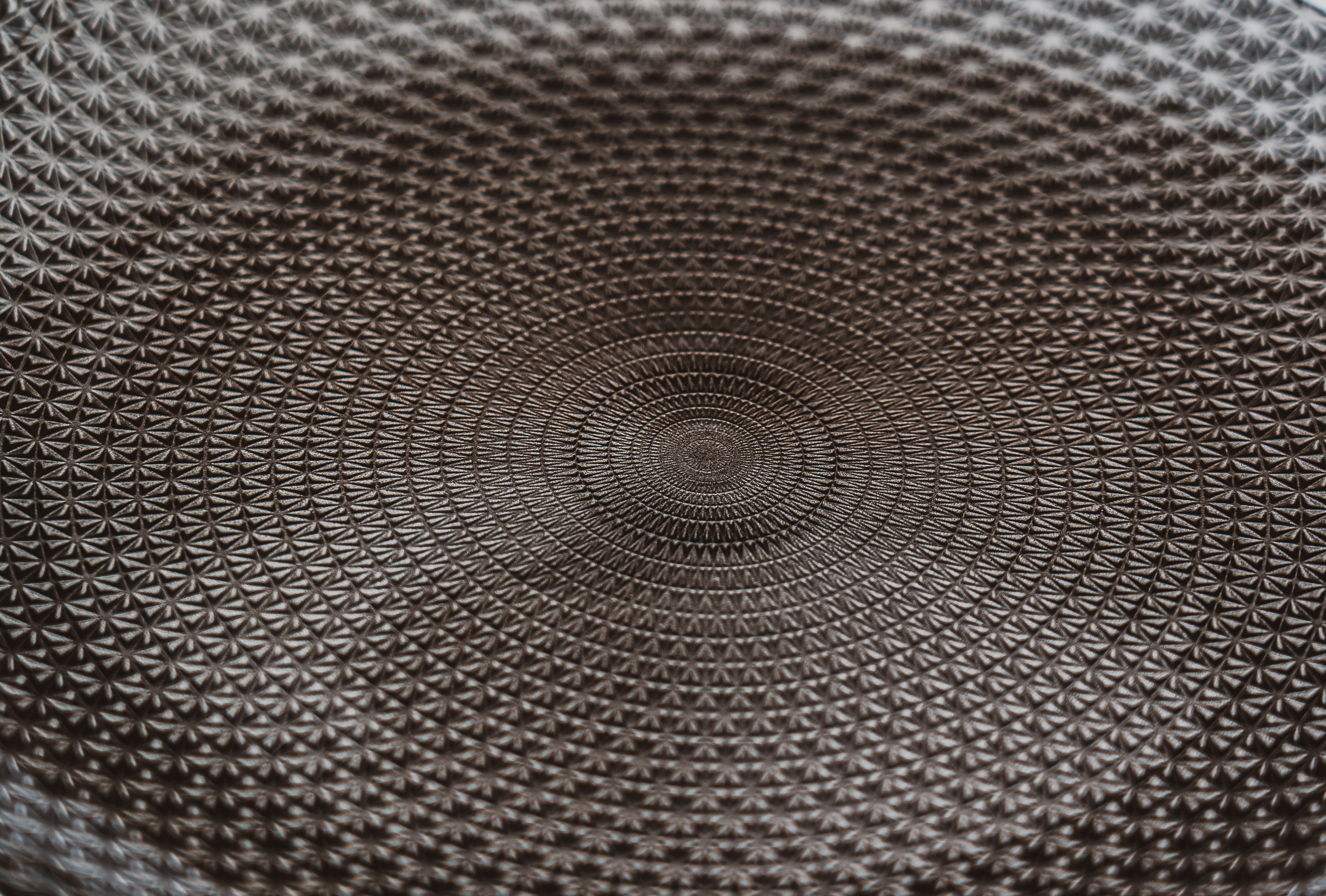# Which is harder calculus or abstract algebra?This is a question that many students may ask themselves when deciding which mathematics subject to study in college. Both Calculus and Abstract Algebra are challenging subjects that require a student’s full commitment and dedication. However, both subjects have different levels of difficulty and may require a different set of skills to master. This article aims to answer the question: Which is harder calculus or abstract algebra?

## FAQ:

### What is Calculus?Calculus is a branch of mathematics that deals with rates of change and slopes of curves. Students usually take Calculus courses in their senior years of high school or in college. The subject mainly consists of two branches: Differential Calculus and Integral Calculus.

### What is Abstract Algebra?Abstract Algebra is a branch of mathematics that studies algebraic structures like groups, rings, and fields. It is considered an advanced subject and is usually offered as an elective in college or graduate school.

### Which is easier Calculus or Abstract Algebra?It depends on the student. Some students may find Calculus easier because it is more concrete and has practical applications in fields like physics and engineering. Other students may find Abstract Algebra easier because it uses abstract concepts and logic rather than calculations. However, a student’s background and previous mathematics experience may also play a role in determining which subject is easier for them.

### Which is harder Calculus or Abstract Algebra?Abstract Algebra is considered harder than Calculus, mainly because it requires a higher level of abstraction and a different way of thinking about mathematics. Abstract Algebra is more concerned with concepts like groups, rings, and fields, which are difficult to visualize and require a lot of abstract thinking. On the other hand, Calculus deals with more concrete concepts like limits, derivatives, and integrals, which have practical applications.

## Comparison between Calculus and Abstract Algebra:

### Level of abstraction:Abstract Algebra requires a higher level of abstraction compared to Calculus. It deals with algebraic structures like groups, rings, and fields, which are difficult to visualize and require a lot of abstract thinking. On the other hand, Calculus deals with more concrete concepts like limits, derivatives, and integrals, which have practical applications and are easier to visualize.

### Level of difficulty:Abstract Algebra is considered a harder subject compared to Calculus. This is mainly because it requires a different way of thinking about mathematics and involves more abstract concepts like groups, rings, and fields. On the other hand, Calculus deals with more concrete concepts and has practical applications in fields like physics and engineering.

### Skills required:Abstract Algebra requires strong logical and abstract thinking skills. It involves understanding complex concepts, theorems, and proofs. On the other hand, Calculus requires strong analytical and computational skills.

## Conclusion:Which is harder Calculus or Abstract Algebra? The answer depends on the student, their background, and previous mathematics experience. However, in general, Abstract Algebra is considered a harder subject compared to Calculus. It requires a higher level of abstraction, strong logical and abstract thinking skills, and a different way of thinking about mathematics. On the other hand, Calculus deals with more concrete concepts and has practical applications in fields like physics and engineering.